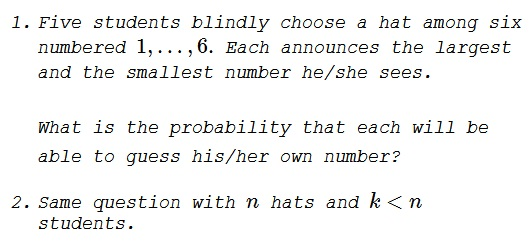# Guessing Hat Numbers

### Problem### Solution to Question 1

First, let's solve a more straightforward problem:

1. Five students blindly choose a hat among six numbered $1,\ldots,6.$ Each announces the largest and the smallest number he/she sees.

What is the probability that each will be able to deduce his/her own number?

As there are six hats and five students, exactly one of the hats is bound to be left over. So the sample space for the problem consists of six elements, which cold be he omitted elements or the sequences of numbers on the hats that the students were wearing.

As a general remark, if the selected hats bear numbers $a_1\lt a_2\lt a_3\lt a_4\lt a_5,$ then the numbers $a_1,a_2,a_4,a_5$ will be always called out. (For example, $a_4$ will be called by the wearer of the hat with $a_5.)$ The question is whether number $a_3$ can be deduced with certainty. This will be the case when $a_4-a_2=2,$ i.e., when $a_3$ is tight between $a_2$ and $a_4.$ This will happen, if the omitted number is one of $1,2,5,6;$ the ambiguity will occur when either $3$ or $4$ is left over.

Thus, every student will be able to deduce his/her number in four out of six cases, giving the probability of the correct deduction at $\displaystyle \frac{4}{6}=\frac{2}{3}.$

For the question where students need to guess their numbers, at least four of them will be always able to deduce theirs, i.e., to guess them with the probability of $1.$ In four out of six cases, the remaining person will also be able to deduce his/her number. In two out of six cases the wearer of $a_3$ will have $1:1$ chance to make a correct guess. The total probability then comes to:

$\displaystyle \frac{4}{6}\cdot 1+\frac{2}{6}\cdot\frac{1}{2}=\frac{5}{6}.$

### Solution to Question 2

As in the solution of the first problem, we first look in the case where all the students may or may not deduce the numbers on their hats. We consider $n\ge 6$ and, for $k,$ several cases. Let $P$ be the sought probability.

If $k=1,$ $P=0$ since a single fellow has no way to deduce the number of his/her hat.
If $k=2,$ $P=1$ because two students call out the other's number.
If $k=3$ or $k=4,$ $P=1$ because all numbers will be eventually called out, and each student may pick the number he/she does not see.

So, we focus on $k\gt 4.$ Let the numbers on the hats, in the increasing sequence, be

$a_1\lt a_2\lt \ldots\lt a_{k-1}\lt a_{k}.$

Observe that the numbers $a_1$ and $a_k$ will be called out $k-1$ times each. Numbers $a_2$ and $a_{k-1}$ will be called out just once. If all $k$ numbers are consecutive, i.e., if the above sequence has no gaps then, as in the case $k=3$ or $k=4$ above, each of the students will be able to deduce his/her number. It will be the one he/she does not see.

If there are gaps, some of the students won't be able to deduce their numbers.

The gaps are absent if $a_{k-1}-a_2=k-3$ so that between $a_2$ and $a_{k-1}$ there's enough room for exactly $k-4$ numbers. For $a_2$ we must have $2\le a_2\le n-k+2;$ if $a_2=i\ge 2,$ for $a_1$ there are $i-1$ choices and for $a_k,$ $n-k-i+3$ choices. So, the total number of sequences that allow the students to surmise their hat number is

\displaystyle \begin{align} &\sum_{i=2}^{n-k+2}(i-1)(n-k-i+3)\\ &\qquad\qquad=\sum_{i=2}^{n-k+2}(-i^2+(n-k+4)i-(n-k+3))\\ &\qquad\qquad=-\frac{(n-k+2)(n-k+3)(2n-2k+5)}{6}\\ &\qquad\qquad\qquad+\frac{(n-k+4)(n-k+2)(n-k+3)}{2}-(n-k+2)(n-k+3)\\ &\qquad\qquad=(n-k+2)(n-k+3)\left[-\frac{1}{3}n+\frac{1}{3}k-\frac{5}{6}+\frac{1}{2}n-\frac{1}{2}k+2-1\right]\\ &\qquad\qquad=\frac{(n-k+3)(n-k+2)(n-k+1)}{6}={n-k+3\choose 3}. \end{align}

It follows that the probability of all the students being able to deduce their hat number is

$\displaystyle P=\frac{n-k+3\choose 3}}{n\choose k}}$

Now let's turn to the "guessing" problem. The latest formula is the key. Let $j$ be the total length of the gaps in the sequence $a_2\lt\ldots\lt a_{k-1}.$ $1\le j\le n-k.$ This is the same as the number of students that have no choice but to make a guess. For every $j,$ there are

$\displaystyle {n-(k+j)+3\choose 3}{k-4+j\choose j}$

such sequences making the total equal

$\displaystyle \sum_{j=1}^{n-k}{n-(k+j)+3\choose 3}{k-4+j\choose j}.$

In itself, this sum does not make much sense because, for different $j,$ the probabilities of guessing right differ; in every case they are $(j+1)^{\,-j}.$ However, the total can be verified manually. For example, as we saw, for $n=6$ and $k=5,$ the only possible value for $j$ is $1,$ so we have

$\displaystyle {n-(k+j)+3\choose 3}{k-4+j\choose j}={3\choose 3}{2\choose 1}=2,$

as expected. For $n=7$ and $k=5,$ there are two possibilities: $j=1$ and $j=2.$ The formula gives

$\displaystyle {4\choose 3}{2\choose 1}+{3\choose 3}{3\choose 2}=4\cdot 2+1\cdot 3=11.$

Here are all eleven cases (asterisk denotes the omitted numbers that ought to be guessed):

$\begin{array}{ccccccccc} 1&2&3^*&*&*&6&7&&(j=2)\\ 1&2&*&4^*&*&6&7&&(j=2)\\ 1&2&*&*&5^*&6&7&&(j=2)\\ 1&-&3&4^*&*&6&7&&(j=1)\\ 1&-&3&*&5^*&6&7&&(j=1)\\ -&2&3&4^*&*&6&7&&(j=1)\\ -&2&3&*&5^*&6&7&&(j=1)\\ 1&2&3^*&*&5&-&7&&(j=1)\\ 1&2&*&4^*&5&-&7&&(j=1)\\ 1&2&3^*&*&5&6&-&&(j=1)\\ 1&2&*&4^*&5&6&-&&(j=1)\\ \end{array}$

The general formula for the probability of all students being able to guess correctly appears to be

$\displaystyle P=\frac{n-k+3\choose 3}+\sum_{j=1}^{n-k}(j+1)^{\,-j}{n-(k+j)+3\choose 3}{k-4+j\choose j}}{n\choose k}}$

### Acknowledgment

The problem is based on problem 112 from A Mathematical Orchard by M. I. Krusemeyer, G. T. Gilbert, L. C. Larson (MAA, 2012).